# 风化花岗岩地层隧道变形与施工技术研究Research on Deformation Characteristics and Construction Technology of Weathered Granite Formation Tunnel

• 全文下载: PDF(1315KB)    PP.11-19   DOI: 10.12677/OJTT.2019.81002
• 下载量: 104  浏览量: 198

Based on the measured surrounding rock stress, deformation and support structure stress in the weathered rock formation, combined with analytical formula calculation and numerical simulation, from the surrounding rock loose circle radius, support structure deformation and force, this paper evaluated surrounding rock stability and support effect. The results show that the surrounding rock conditions of the exit section of the Yuliao tunnel are better, the maximum deformation is 7.71 mm, and the thickness of the loose roof of the arch is 3.1 m. The thickness of the loose circle at the arch waist and the arch shoulder is more than 4.0 m. The analysis reflects that the bolts in the loose area of the surrounding rock are subjected to large forces. The stress characteristics of the supporting structure indicate that the additional load transmitted to the supporting structure due to the large deformation of the surrounding rock at the vault and the arch causes the force increase. The numerical calculation results show that there is a significant difference in the deformation of the bolts installed in different processes. Based on the deformation law and the force analysis results of supporting structure, the construction measures and support structure optimization suggestions are proposed.

1. 引言

2. 渔寮隧道工程概况Table 1. The basic information about Yuliao tunnel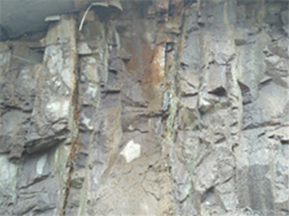Figure 1. Geology of the face in ZK368 + 248

3. 风化花岗岩地层隧道变形特征

3.1. 现场监测数据分析

3.1.1. 拱顶下沉、洞周收敛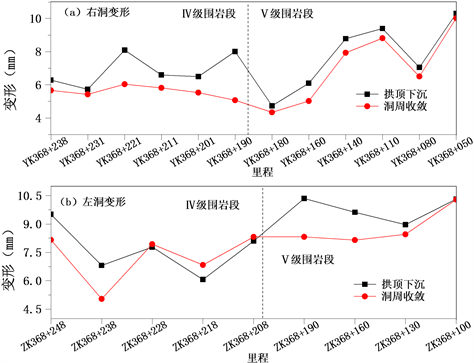Figure 2. Vaulting down and cave convergence of exit section

3.1.2. 拱顶下沉、洞周收敛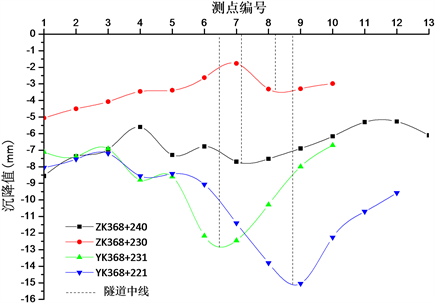Figure 3. Settlement value of different mileage surface in the exit section

$S\left(y\right)={S}_{\mathrm{max}}\mathrm{exp}\left[\frac{-{y}^{2}}{2{i}^{2}}\right]$ (1)

$y={y}_{0}+A\mathrm{exp}\left[-0.5\ast \frac{{\left(x-{x}_{c}\right)}^{2}}{{w}^{2}}\right]$ (2)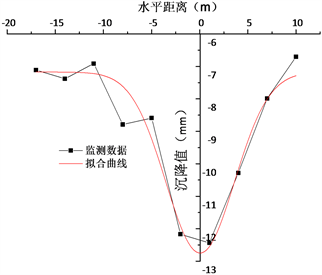(a) YK328+231地表沉降值拟合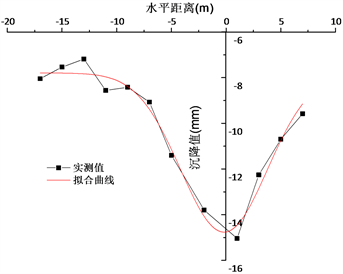(b) YK328 + 221地表沉降值拟合

Figure 4. Surface subsidence in YK328 + 221 and YK328 + 231

YK368 + 231、YK368 + 221：的回归模型依次为：

$y=-7.17-5.59\ast \mathrm{exp}\left[-0.5\ast {\left(\frac{x-0.0367}{3.57}\right)}^{2}\right]$ (3)

$y=-7.79-6.97*\mathrm{exp}\left[-0.5*{\left(\frac{x+0.189}{3.97}\right)}^{2}\right]$ (4)

3.2. 数值模拟分析

3.2.1. 计算模型的建立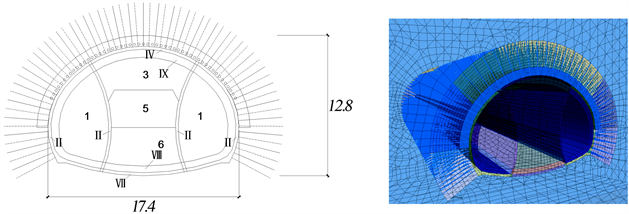Figure 5. Tunnel size (unit: m) and tunnel model

3.2.2. 计算参数确定Table 2. The basic information about Yuliao tunnel

$E=\left({E}_{0}{S}_{0}+E{}_{G}S{}_{G}+{E}_{C}{S}_{C}\right)/S$ (5)

3.2.3. 计算结果分析(a) 隧道围岩竖向变形云图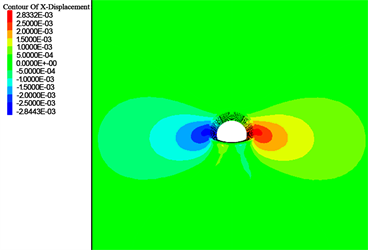(b) 隧道围岩横向变形云图

Figure 6. Tunnel surrounding rock deformation contour (unit: m)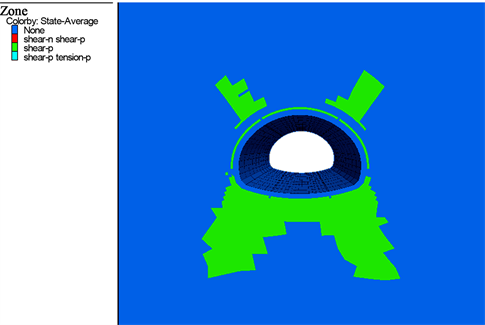Figure 7. Tunnel surrounding rock plastic zone (unit: m)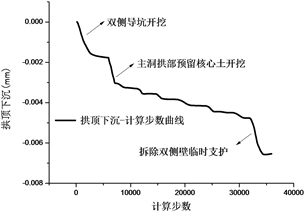(a) 拱顶下沉——计算步数曲线(b) 拱腰收敛——计算步数曲线

Figure 8. Tunnel deformation—calculating step curve

4. 结论

1) 渔寮隧道出口段处于风化花岗岩地层中，围岩级别由洞口向内逐步自V级往IV级过渡，在隧道变形沿纵向的变化中能够反映出来，V级围岩区段的隧洞变形量比IV级围岩区段的隧洞要大，IV、V级围岩的分界线也在隧道变形中能反映出来。

2) 渔寮隧道出口段右洞地表形成了明显的沉降槽，根据实测地表沉降数据，基于Peck理论，拟合得到地表沉降预测模型表达式，据此可推测出不同水平位置处的地表沉降量。

3) 数值模拟结果显示：双侧壁导坑临时支护的拆除以及主洞拱部岩体开挖是引起隧道围岩变形的主要工况；开挖导致拱肩、仰拱和拱脚处出现较大的塑性区，是施工中重点控制变形的位置。

4) 在渔寮隧道出口段的风化花岗岩地层施工中，围岩条件较差的V级围岩段采用长管棚或超前小导管注浆预支护，节理裂隙发育和断层等软弱围岩地段设置超前砂浆锚杆，不同围岩条件采用合理的开挖工法，控制爆破减少围岩扰动和减少超挖，多项施工技术措施控制围岩变形，预防出现地表坍塌、拱顶掉块及变形超限等问题。

  陈洪江. 花岗石类全风化带的工程地质特性[J]. 港工技术, 1995(3): 61-65.  张海洋, 徐文杰, 王永刚. 各向异性软岩隧道围岩蠕变特征及支护方案对比分析[J]. 铁道建筑, 2018, 58(8): 71-74.  任文峰, 高璇, 王星华, 等. 富水全风化花岗岩隧道变形规律与力学特性[J]. 交通运输工程学报, 2013, 13(2): 34-41.  龚彦峰, 张俊儒, 徐向东, 等. 全风化花岗岩富水地层超大断面隧道设计技术[J]. 铁道工程学报, 2015, 32(10): 79-85+92.  周平. 昔格达地层长大铁路隧道变形——灾变特征及失稳机理研究[D]: [硕士学位论文]. 成都: 西南交通大学, 2018.  徐莹, 泽旺四郎. 拉丁拉山全风化花岗岩隧道塌方处治[J]. 公路交通技术, 2017, 33(6): 84-88.  Peck, R.B. (1969) Deep Excavation and Tunneling in Soft Ground. State of the Art Report. 7th International Conference on Soil Mechanics and Foundation Engineering, Mexico City, 225-290.  李术才, 朱维申, 陈卫忠, 等. 弹塑性大位移有限元方法在软岩隧道变形预估系统研究中的应用[J]. 岩石力学与工程学报, 2002, 21(4): 466-470.